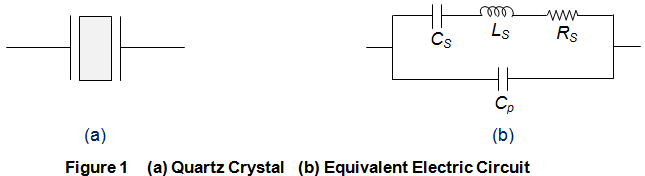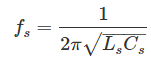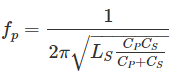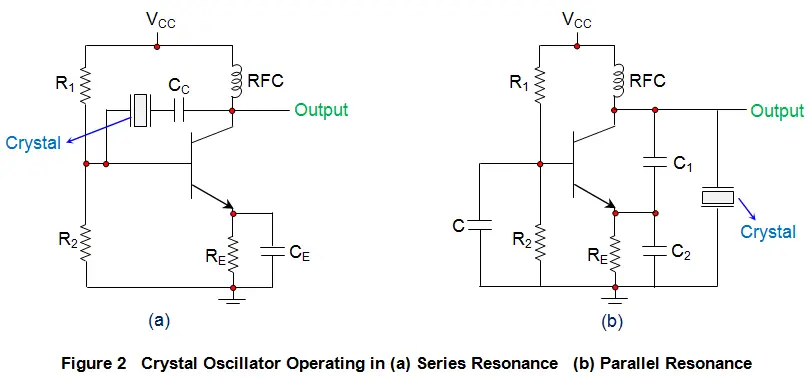# Crystal Oscillator: Circuit, Frequency & Working Principle

Crystal oscillators operate on the principle of inverse piezoelectric effect in which an alternating voltage applied across the crystal surfaces causes it to vibrate at its natural frequency. It is these vibrations which eventually get converted into oscillations.

These oscillators are usually made of Quartz crystal, eventhough other substances like Rochelle salt and Tourmaline exhibit the piezoelectric effect because, quartz is inexpensive, naturally-available and mechanically-strong when compared to others.

In crystal oscillators, the crystal is suitably cut and mounted between two metallic plates as shown by Figure 1a whose electrical equivalent is shown by Figure 1b. In reality, the crystal behaves like a series RLC circuit, formed by the components

1. A low-valued resistor RS
2. A large-valued inductor LS
3. A small-valued capacitor CS

which will be in parallel with the capacitance of its electrodes Cp.Due to the presence of Cp, the crystal will resonate at two different frequencies viz.,

1. Series Resonant Frequency, fs which occurs when the series capacitance CS resonates with the series inductance LS. At this stage, the crystal impedance will be the least and hence the amount of feedback will be the largest. Mathematical expression for the same is given as2. Parallel Resonant frequency, fp which is exhibited when the reactance of the LSCS leg equals the reactance of the parallel capacitor Cp i.e. LS and CS resonate with Cp. At this instant, the crystal impedance will be the highest and thus the feedback will be the least. Mathematically it can be given asThe behaviour of the capacitor will be capacitive both below fS and above fp. However for the frequencies which lie in-between fS and above fp, the crystal’s behavior will be inductive. Further when the frequency becomes equal to parallel resonant frequency fp, then the interaction between LS and Cp would form a parallel tuned LC tank circuit. Hence, a crystal can be viewed as a combination of series and parallel tuned resonance circuits due to which one needs to tune the circuit for any one among these two. Moreover it is to be noted that fp will be higher than fs and the closeness between the two will be decided by the cut and the dimensions of the crystal in-use.

Crystal oscillators can be designed by connecting the crystal into the circuit such that it offers low impedance when operated in series-resonant mode (Figure 2a) and high impedance when operated in anti-resonant or parallel resonant mode (Figure 2b).In the circuits shown, the resistors R1 and R2 form the voltage divider network while the emitter resistor RE stabilizes the circuit. Further, CE (Figure 2a) acts as an AC bypass capacitor while the coupling capacitor CC (Figure 2a) is used to block DC signal propagation between the collector and the base terminals.

Next, the capacitors C1 and C2 form the capacitive voltage divider network in the case of Figure 2b. In addition, there is also a Radio Frequency Coil (RFC) in the circuits (both in Figure 2a and 2b) which offers dual advantage as it provides even the DC bias as well as frees the circuit-output from being affected by the AC signal on the power lines.

On supplying the power to the oscillator, the amplitude of the oscillations in the circuit increases until a point is reached wherein the nonlinearities in the amplifier reduce the loop gain to unity.

Next, on reaching the steady state, the crystal in the feedback loop highly influences the frequency of the operating circuit. Further, here, the frequency will self-adjust so as to facilitate the crystal to present a reactance to the circuit such that the Barkhausen phase requirement is fulfilled.

In general, the frequency of the crystal oscillators will be fixed to be the crystal’s fundamental or characteristic frequency which will be decided by the physical size and shape of the crystal.

However, if the crystal is non-parallel or of non-uniform thickness, then it might resonate at multiple frequencies, resulting in harmonics.

Further, the crystal oscillators can be tuned to either even or odd harmonic of the fundamental frequency, which are called Harmonic and Overtone Oscillators, respectively.

An example of this is the case where the parallel resonance frequency of the crystal is decreased or increased by adding a capacitor or an inductor across the crystal, respectively.

The typical operating range of the crystal oscillators is from 40 KHz to 100 MHz wherein the low frequency oscillators are designed using OpAmps while the high frequency-ones are designed using the transistors (BJTs or FETs).

The frequency of oscillations generated by the circuit is decided by the series resonant frequency of the crystal and will be unaffected by the variations in supply voltage, transistor parameters, etc. As a result, crystal oscillators exhibit a high Q-factor with excellent frequency stability, making them most suitable for high-frequency applications.

However, care should be taken so as to drive the crystal with optimum power only. This is because, if too much power is delivered to the crystal, then the parasitic resonances might be excited in the crystal which leads to the unstable resonant frequency.

Further, even its output waveform might be distorted due to the degradation in its phase noise performance. Moreover, it can even result in the destruction of the device (crystal) due to overheating.

Crystal oscillators are compact in size and are of low cost due to which they are extensively used in electronic warfare systems, communication systems, guidance systems, microprocessors, microcontrollers, space tracking systems, measuring instruments, medical devices, computers, digital systems, instrumentation, phase-locked loop systems, modems, sensors, disk drives, marine systems, telecommunications, engine control systems, clocks, Global Positioning Systems (GPS), cable television systems, video cameras, toys, video games, radio systems, cellular phones, timers, etc.

Want To Learn Faster? 🎓
Get electrical articles delivered to your inbox every week.
No credit card required—it’s 100% free.